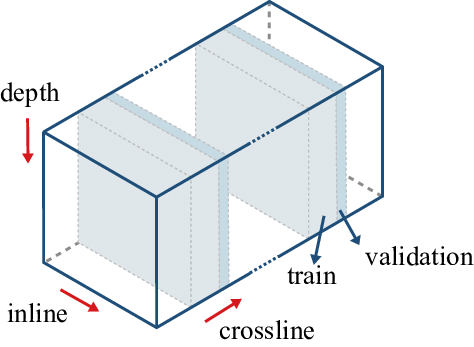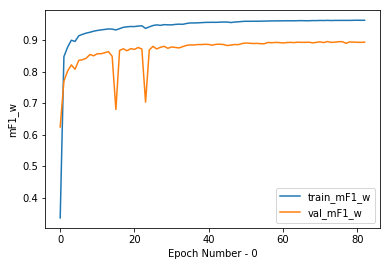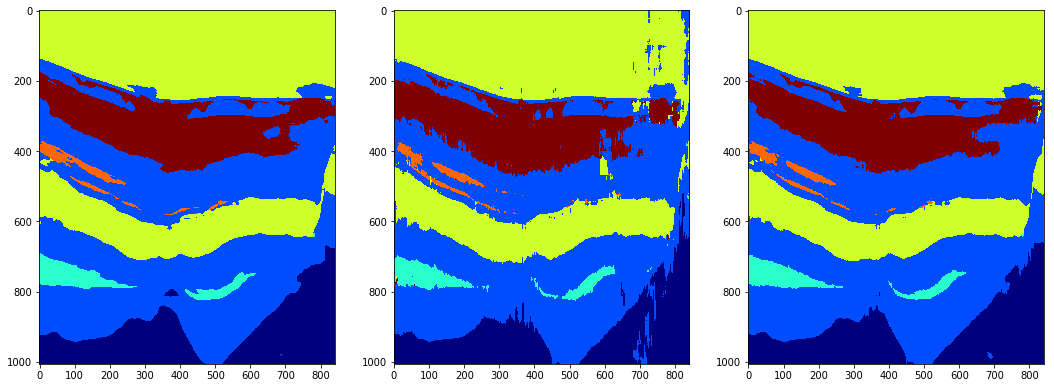# [Explainer] End-to-end solution [F1-Score (weighted) - 0.76]

Solution for 4th round

This notebook presents my solution for this competition. In summary, the process was as follows:

• Split of sections / images for train and validation . For this, in each direction (inline and crossline), the total of sections were divided into 10 groups. From each group, 70% of the first sections were for training and the rest for validation. This to avoid overfitting.
• Creation of patches . These were generated from the sections selected in the split. In order not to lose much information and to be compatible with encoder-decoder networks, 512x512 patches were established. In addition to this, hard data augmentation was applied.
• Training . The network used was a variation of the well-known U-Net. In this proposed version, dilated convolutional layers were used in the bottleneck to keep the context information since the images are relatively large. The stopping criterion was used with respect to the weighted F1-score, where the weight of each class was the inverse of its frequency.
• Inference . Finally, inference was performed in both directions separately, and then an ensembling was applied to give a more stable result.

### Import libraries¶

In :
```import numpy as np
import matplotlib.pyplot as plt
from skimage.util.shape import view_as_windows
from tqdm import tqdm
import random
import os

from keras.utils import Sequence
from keras.callbacks import EarlyStopping, ReduceLROnPlateau, LearningRateScheduler, ModelCheckpoint

# custom functions
from models import AtrousUNet
from metrics import mF1_w
from utils import PlotHistory

from albumentations import (
HorizontalFlip, VerticalFlip,
ShiftScaleRotate, OpticalDistortion, GridDistortion, ElasticTransform,
RandomBrightnessContrast,
IAASharpen, IAAEmboss, Flip, OneOf, Compose, RandomSizedCrop, IAAAffine
)

os.environ["CUDA_VISIBLE_DEVICES"] = "0"
```

# 1. Split¶

For the split, the process shown in the image below was followed, where in this case it is being carried out along the crossline direction. As can be seen, they are divided into groups (10 in our case), where 70% of the first images go to train, and the rest to validation. The same is done in the inline direction.In :
```# paths:
root = 'seismic-facies-identification-challenge/'
save_folder = 'patches1'

# scale factor
min_ = -5184.1055
max_ = 5151.7188

# patch size
ps = 512
step = 100
```
In :
```# loading data
y.shape # Z, X, Y
```
Out:
`(1006, 782, 590)`

### Generate splits texts:¶

In :
```def split_train_val(iline, xline, loader_type='section', n_groups=10, per_val=0.3):
"""
Create txt files to split inline and crossline 2D sections in training and validation
"""

vert_locations = range(0, iline-n_groups, n_groups)
horz_locations = range(0, xline-n_groups, n_groups)

p_tr_list = []
p_vl_list = []
for i in vert_locations:
vert_slides = np.arange(i, i+n_groups)

aux = list(vert_slides[:-int(per_val*n_groups)])
p_tr_list += ['i_' + str(ii) for ii in aux if ii < iline]

aux = list(vert_slides[-int(per_val*n_groups):])
p_vl_list += ['i_' + str(ii) for ii in aux if ii < iline]

random.shuffle(p_tr_list)
random.shuffle(p_vl_list)

aux1 = []
aux2 = []
for j in horz_locations:
horz_slides = np.arange(j, j+n_groups)

aux = list(horz_slides[:-int(per_val*n_groups)])
aux1 += ['x_' + str(jj) for jj in aux if jj < xline]

aux = list(horz_slides[-int(per_val*n_groups):])
aux2 += ['x_' + str(jj) for jj in aux if jj < xline]

random.shuffle(aux1)
random.shuffle(aux2)

p_tr_list+=aux1
p_vl_list+=aux2

path_splits = '{}/splits'.format(root)

if not os.path.exists(path_splits):
os.makedirs(path_splits)

file_object = open(os.path.join(path_splits, loader_type + '_train.txt'), 'w')
file_object.write('\n'.join(p_tr_list))
file_object.close()

file_object = open(os.path.join(path_splits, loader_type + '_val.txt'), 'w')
file_object.write('\n'.join(p_vl_list))
file_object.close()
```
In :
```_, iline, xline = y.shape
```

# 2. Patches Generation¶

Although there are scientific articles that demonstrate the superiority of using slices over 2D patches in volumes of data, in this case, the slices are relatively large with respect to the classes with less presence. That is why 512x512 patches were generated in this process to avoid losing context information. All of them are saved as .npy file.

In :
```# scaling
X = (X - min_)/(max_ - min_)

y.shape
```
Out:
`(1012, 812, 612)`
In :
```def makePatches(split='train'):
"""
Save each patch as .npy file
"""

os.makedirs("{}/{}/input".format(save_folder, split))
os.makedirs("{}/{}/label".format(save_folder, split))

path = os.path.join(root, 'splits', 'section2_' + split + '.txt')
patch_list = tuple(open(path, 'r'))

data = {}
data['i'] = []
data['x'] = []

for indexes in patch_list:
direction, number = indexes.split(sep='_')
if direction == 'i':
data['i'].append(int(number))
elif direction == 'x':
data['x'].append(int(number))

X_train = []
y_train = []

# direction i
subX = X[:, data['i'], :]
suby = y[:, data['i'], :]

for i in tqdm(range(suby.shape)):
img  = subX[:,i]

splitsX = view_as_windows(img, (ps, ps), step=step)
splitsy = view_as_windows(mask, (ps, ps), step=step)

ni, nj, _, _ = splitsy.shape

X_train.append(np.reshape(splitsX, (ni*nj, ps, ps)))
y_train.append(np.reshape(splitsy, (ni*nj, ps, ps)))

# direction x
subX = X[:, :, data['x']]
suby = y[:, :, data['x']]

for i in tqdm(range(suby.shape)):
img  = subX[:,:,i]

splitsX = view_as_windows(img, (ps, ps), step=step)
splitsy = view_as_windows(mask, (ps, ps), step=step)

ni, nj, _, _ = splitsy.shape

X_train.append(np.reshape(splitsX, (ni*nj, ps, ps)))
y_train.append(np.reshape(splitsy, (ni*nj, ps, ps)))

X_train = np.vstack(X_train)
y_train = np.vstack(y_train)

# saving
for i in tqdm(range(len(X_train))):
np.save('{}/{}/input/{}.npy'.format(save_folder, split, i), X_train[i])
np.save('{}/{}/label/{}.npy'.format(save_folder, split, i), y_train[i])
```
In :
```makePatches(split='train')
makePatches(split='val')
```
```  0%|          | 0/546 [00:00<?, ?it/s]/usr/local/lib/python3.5/dist-packages/skimage/util/shape.py:246: RuntimeWarning: Cannot provide views on a non-contiguous input array without copying.
warn(RuntimeWarning("Cannot provide views on a non-contiguous input "
100%|██████████| 546/546 [00:03<00:00, 154.35it/s]
100%|██████████| 406/406 [00:04<00:00, 88.32it/s]
100%|██████████| 16296/16296 [01:16<00:00, 213.64it/s]
100%|██████████| 234/234 [00:01<00:00, 151.06it/s]
100%|██████████| 174/174 [00:01<00:00, 89.40it/s]
100%|██████████| 6984/6984 [00:30<00:00, 230.63it/s]
```
In :
```# clean memory
del X, y
```

# 3. Training¶

The neural network used is a modified version of U-Net with its main difference being the bottleneck. For more details, see its implementation in Keras. For loss, the categorical cross-entropy loss seems to give good results.

In :
```idd=10
model_name = "atrous_unet"
f1 = 16
type_aug = "aug10"

bs = 32
_epochs = 100
lossf = 'categorical_crossentropy'
```

### Generator¶

Since a large number of patches were generated, it was decided to use a generator to avoid filling the memory. Data augmentation was implemented within the generator.

In :
```def one_hot(y):
"""Convert images to one-hot format."""
return np.eye(6)[y]
```
In :
```class DataGenerator(Sequence):
'Generates data for Keras'
def __init__(self, split='train', batch_size=32, shuffle=True, aug='all'):

self.split = split
self.batch_size = batch_size
self.aug = aug
self.shuffle = shuffle

x = os.listdir('{}/{}/input/'.format(save_folder, split))
self.list_IDs = [i for i in range(len(x))]

self.on_epoch_end()

def __len__(self):
'Denotes the number of batches per epoch'
return int(np.floor(len(self.list_IDs) / self.batch_size))

def __getitem__(self, index):
'Generate one batch of data'
# Generate indexes of the batch
indexes = self.indexes[index*self.batch_size:(index+1)*self.batch_size]

# Find list of IDs
list_IDs_temp = [self.list_IDs[k] for k in indexes]

# Generate data
X, y = self.__data_generation(list_IDs_temp)

return X, y

def on_epoch_end(self):
self.indexes = np.arange(len(self.list_IDs))
if self.shuffle == True:
np.random.shuffle(self.indexes)

def __data_generation(self, list_IDs_temp):
'Generates data containing batch_size samples' # X : (n_samples, *dim, n_channels)
# Initialization
X = np.empty((self.batch_size, 512, 512, 1))
y = np.empty((self.batch_size, 512, 512, 6), dtype=int)

# Generate data
for i, ID in enumerate(list_IDs_temp):

yy = np.load('patches_v2/{}/label/{}.npy'.format(self.split, ID)).astype(int) - 1
yy = one_hot(yy)

if self.aug:
X_aug, y_aug = self.augmentation(xx, yy)

# Store sample
X[i,:,:,0] = X_aug

# Store class
y[i,] = y_aug

return X, y

def augmentation(self, X, y):

if self.aug == type_aug:
aug = OneOf([
ShiftScaleRotate(shift_limit=0.0625, scale_limit=0.2, rotate_limit=45, p=1),
IAASharpen(p=1),
IAAEmboss(p=1),
RandomBrightnessContrast(p=1),
Compose([VerticalFlip(p=0.5), HorizontalFlip(p=0.5)]),
RandomSizedCrop(p=1, min_max_height=[384, 500], height=512, width=512),
IAAAffine(p=1, translate_percent=0.3),
ElasticTransform(p=1, alpha=400, sigma=400 * 0.05, alpha_affine=400 * 0.03),
GridDistortion(p=1),
OpticalDistortion(p=1)
], p=0.91)

```
In :
```train_gen = DataGenerator('train', batch_size=bs, aug=type_aug)
val_gen = DataGenerator('val', batch_size=bs, shuffle=False)
```

### Modeling¶

In :
```model = AtrousUNet(n_classes=6, filters=f1, n_block=4, BN=True)
model.summary()
```
```__________________________________________________________________________________________________
Layer (type)                    Output Shape         Param #     Connected to
==================================================================================================
input_4 (InputLayer)            (None, None, None, 1 0
__________________________________________________________________________________________________
conv2d_70 (Conv2D)              (None, None, None, 1 160         input_4
__________________________________________________________________________________________________
batch_normalization_49 (BatchNo (None, None, None, 1 64          conv2d_70
__________________________________________________________________________________________________
activation_49 (Activation)      (None, None, None, 1 0           batch_normalization_49
__________________________________________________________________________________________________
conv2d_71 (Conv2D)              (None, None, None, 1 2320        activation_49
__________________________________________________________________________________________________
batch_normalization_50 (BatchNo (None, None, None, 1 64          conv2d_71
__________________________________________________________________________________________________
activation_50 (Activation)      (None, None, None, 1 0           batch_normalization_50
__________________________________________________________________________________________________
max_pooling2d_13 (MaxPooling2D) (None, None, None, 1 0           activation_50
__________________________________________________________________________________________________
conv2d_72 (Conv2D)              (None, None, None, 3 4640        max_pooling2d_13
__________________________________________________________________________________________________
batch_normalization_51 (BatchNo (None, None, None, 3 128         conv2d_72
__________________________________________________________________________________________________
activation_51 (Activation)      (None, None, None, 3 0           batch_normalization_51
__________________________________________________________________________________________________
conv2d_73 (Conv2D)              (None, None, None, 3 9248        activation_51
__________________________________________________________________________________________________
batch_normalization_52 (BatchNo (None, None, None, 3 128         conv2d_73
__________________________________________________________________________________________________
activation_52 (Activation)      (None, None, None, 3 0           batch_normalization_52
__________________________________________________________________________________________________
max_pooling2d_14 (MaxPooling2D) (None, None, None, 3 0           activation_52
__________________________________________________________________________________________________
conv2d_74 (Conv2D)              (None, None, None, 6 18496       max_pooling2d_14
__________________________________________________________________________________________________
batch_normalization_53 (BatchNo (None, None, None, 6 256         conv2d_74
__________________________________________________________________________________________________
activation_53 (Activation)      (None, None, None, 6 0           batch_normalization_53
__________________________________________________________________________________________________
conv2d_75 (Conv2D)              (None, None, None, 6 36928       activation_53
__________________________________________________________________________________________________
batch_normalization_54 (BatchNo (None, None, None, 6 256         conv2d_75
__________________________________________________________________________________________________
activation_54 (Activation)      (None, None, None, 6 0           batch_normalization_54
__________________________________________________________________________________________________
max_pooling2d_15 (MaxPooling2D) (None, None, None, 6 0           activation_54
__________________________________________________________________________________________________
conv2d_76 (Conv2D)              (None, None, None, 1 73856       max_pooling2d_15
__________________________________________________________________________________________________
batch_normalization_55 (BatchNo (None, None, None, 1 512         conv2d_76
__________________________________________________________________________________________________
activation_55 (Activation)      (None, None, None, 1 0           batch_normalization_55
__________________________________________________________________________________________________
conv2d_77 (Conv2D)              (None, None, None, 1 147584      activation_55
__________________________________________________________________________________________________
batch_normalization_56 (BatchNo (None, None, None, 1 512         conv2d_77
__________________________________________________________________________________________________
activation_56 (Activation)      (None, None, None, 1 0           batch_normalization_56
__________________________________________________________________________________________________
max_pooling2d_16 (MaxPooling2D) (None, None, None, 1 0           activation_56
__________________________________________________________________________________________________
conv2d_78 (Conv2D)              (None, None, None, 2 295168      max_pooling2d_16
__________________________________________________________________________________________________
conv2d_79 (Conv2D)              (None, None, None, 2 590080      conv2d_78
__________________________________________________________________________________________________
conv2d_80 (Conv2D)              (None, None, None, 2 590080      conv2d_79
__________________________________________________________________________________________________
conv2d_81 (Conv2D)              (None, None, None, 2 590080      conv2d_80
__________________________________________________________________________________________________
conv2d_82 (Conv2D)              (None, None, None, 2 590080      conv2d_81
__________________________________________________________________________________________________
conv2d_83 (Conv2D)              (None, None, None, 2 590080      conv2d_82
__________________________________________________________________________________________________
conv2d_79
conv2d_80
conv2d_81
conv2d_82
conv2d_83
__________________________________________________________________________________________________
conv2d_transpose_13 (Conv2DTran (None, None, None, 1 295040      add_4
__________________________________________________________________________________________________
concatenate_13 (Concatenate)    (None, None, None, 2 0           conv2d_transpose_13
activation_56
__________________________________________________________________________________________________
conv2d_84 (Conv2D)              (None, None, None, 1 295040      concatenate_13
__________________________________________________________________________________________________
batch_normalization_57 (BatchNo (None, None, None, 1 512         conv2d_84
__________________________________________________________________________________________________
activation_57 (Activation)      (None, None, None, 1 0           batch_normalization_57
__________________________________________________________________________________________________
conv2d_85 (Conv2D)              (None, None, None, 1 147584      activation_57
__________________________________________________________________________________________________
batch_normalization_58 (BatchNo (None, None, None, 1 512         conv2d_85
__________________________________________________________________________________________________
activation_58 (Activation)      (None, None, None, 1 0           batch_normalization_58
__________________________________________________________________________________________________
conv2d_transpose_14 (Conv2DTran (None, None, None, 6 73792       activation_58
__________________________________________________________________________________________________
concatenate_14 (Concatenate)    (None, None, None, 1 0           conv2d_transpose_14
activation_54
__________________________________________________________________________________________________
conv2d_86 (Conv2D)              (None, None, None, 6 73792       concatenate_14
__________________________________________________________________________________________________
batch_normalization_59 (BatchNo (None, None, None, 6 256         conv2d_86
__________________________________________________________________________________________________
activation_59 (Activation)      (None, None, None, 6 0           batch_normalization_59
__________________________________________________________________________________________________
conv2d_87 (Conv2D)              (None, None, None, 6 36928       activation_59
__________________________________________________________________________________________________
batch_normalization_60 (BatchNo (None, None, None, 6 256         conv2d_87
__________________________________________________________________________________________________
activation_60 (Activation)      (None, None, None, 6 0           batch_normalization_60
__________________________________________________________________________________________________
conv2d_transpose_15 (Conv2DTran (None, None, None, 3 18464       activation_60
__________________________________________________________________________________________________
concatenate_15 (Concatenate)    (None, None, None, 6 0           conv2d_transpose_15
activation_52
__________________________________________________________________________________________________
conv2d_88 (Conv2D)              (None, None, None, 3 18464       concatenate_15
__________________________________________________________________________________________________
batch_normalization_61 (BatchNo (None, None, None, 3 128         conv2d_88
__________________________________________________________________________________________________
activation_61 (Activation)      (None, None, None, 3 0           batch_normalization_61
__________________________________________________________________________________________________
conv2d_89 (Conv2D)              (None, None, None, 3 9248        activation_61
__________________________________________________________________________________________________
batch_normalization_62 (BatchNo (None, None, None, 3 128         conv2d_89
__________________________________________________________________________________________________
activation_62 (Activation)      (None, None, None, 3 0           batch_normalization_62
__________________________________________________________________________________________________
conv2d_transpose_16 (Conv2DTran (None, None, None, 1 4624        activation_62
__________________________________________________________________________________________________
concatenate_16 (Concatenate)    (None, None, None, 3 0           conv2d_transpose_16
activation_50
__________________________________________________________________________________________________
conv2d_90 (Conv2D)              (None, None, None, 1 4624        concatenate_16
__________________________________________________________________________________________________
batch_normalization_63 (BatchNo (None, None, None, 1 64          conv2d_90
__________________________________________________________________________________________________
activation_63 (Activation)      (None, None, None, 1 0           batch_normalization_63
__________________________________________________________________________________________________
conv2d_91 (Conv2D)              (None, None, None, 1 2320        activation_63
__________________________________________________________________________________________________
batch_normalization_64 (BatchNo (None, None, None, 1 64          conv2d_91
__________________________________________________________________________________________________
activation_64 (Activation)      (None, None, None, 1 0           batch_normalization_64
__________________________________________________________________________________________________
conv2d_92 (Conv2D)              (None, None, None, 6 102         activation_64
==================================================================================================
Total params: 4,522,662
Trainable params: 4,520,742
Non-trainable params: 1,920
__________________________________________________________________________________________________
```
In :
```lr = 1e-3

model.compile(optimizer=opt,
loss = lossf,
metrics=['acc', mF1_w])
```

### Train Model¶

In :
```path_res = '/scratch/parceirosbr/maykol.trinidad/Seismic_Challenge/res'
name_weight = '{}_{}_{}_f{}_tmp.hdf5'.format(model_name, idd, type_aug, f1)
filepath = os.path.join(path_res, name_weight)
stopPatience = 10
```
In :
```modelCheck = ModelCheckpoint(filepath,verbose=1, save_best_only=True, save_weights_only=True, period=1, monitor="val_mF1_w", mode='max')
earlystopper = EarlyStopping(patience=stopPatience, verbose=1, monitor="val_mF1_w", mode='max')
reduce_lr = ReduceLROnPlateau(factor=0.5, patience=stopPatience//2, min_lr=0.0000005, verbose=1, monitor="val_mF1_w", mode='max')
cb = [earlystopper,reduce_lr,modelCheck]
```
In [ ]:
```hs = model.fit_generator(train_gen, epochs=_epochs,
steps_per_epoch=len(train_gen),
callbacks=cb,
validation_data=val_gen, validation_steps=len(val_gen),
verbose=1, workers=0)
```
```WARNING:tensorflow:From /usr/local/lib/python3.5/dist-packages/tensorflow/python/ops/math_ops.py:3066: to_int32 (from tensorflow.python.ops.math_ops) is deprecated and will be removed in a future version.
Instructions for updating:
WARNING:tensorflow:From /usr/local/lib/python3.5/dist-packages/tensorflow/python/ops/math_grad.py:102: div (from tensorflow.python.ops.math_ops) is deprecated and will be removed in a future version.
Instructions for updating:
Deprecated in favor of operator or tf.math.divide.
Epoch 1/10
177/509 [=========>....................] - ETA: 23:15 - loss: 1.0351 - acc: 0.6384 - mF1_w: 0.1113```
In :
```PlotHistory(hs, 'mF1_w')
```In :
```max(hs.history["val_mF1_w"])
```
Out:
`0.8949835784794598`

# 4. Inference¶

As the model was trained using patches in both directions, it was decided to also infer in each direction and then perform an ensembling. Since many images are overlaid, it was also created a count matrix that counts the number of times a pixel has been overlaid. In this way, when dividing the cumulative prediction matrix over the count matrix, the range was kept between 0 and 1.

In :
```# load the best model
```
In :
```step = 100
```

### X axis¶

In :
```test2 = np.load(os.path.join(root, 'data_test_2.npz' ))['data']
test2.shape
```
Out:
`(1006, 334, 841)`
In [ ]:
```#padding
```
In :
```# scaling
```
In :
```pred_pad   = np.zeros(test2_pad.shape + (6,))

for iin in tqdm(range(test2.shape)):

splits = view_as_windows(section, (ps, ps), step=step)
ni, nj, _, _ = splits.shape

ii = 0
for i in range(ni):
jj = 0
for j in range(nj):
split_ij = np.expand_dims(splits[i,j], axis=[0,-1])
jj+=step
ii+=step
```
```  0%|          | 0/334 [00:00<?, ?it/s]/usr/local/lib/python3.5/dist-packages/skimage/util/shape.py:246: RuntimeWarning: Cannot provide views on a non-contiguous input array without copying.
warn(RuntimeWarning("Cannot provide views on a non-contiguous input "
100%|██████████| 334/334 [04:35<00:00,  1.21it/s]
```
In :
```# normalize results
```

### Y axis¶

In :
```data = np.concatenate([
np.concatenate([
], axis=2),
test2
], axis=1)
data.shape
```
Out:
`(1006, 1116, 841)`
In :
```data = data[:,604:]
data.shape
```
Out:
`(1006, 512, 841)`
In :
```test22_pad = np.pad(data,((0,6),(0,0), (0,0)),"reflect")
```
Out:
`(1012, 512, 841)`
In :
```del data, counts_pad
```
In :
```# scaling
```
In :
```pred_pad2   = np.zeros(test22_pad.shape + (6,))

for xn in tqdm(range(test2.shape)):

splits = view_as_windows(section, (ps, ps), step=step)
ni, nj, _, _ = splits.shape

ii = 0
for i in range(ni):
jj = 0
for j in range(nj):
split_ij = np.expand_dims(splits[i,j], axis=[0,-1])
pred_pad2[ii:(ii+ps) , jj:(jj+ps), xn , :] += model.predict(split_ij)
counts_pad2[ii:(ii+ps) , jj:(jj+ps), xn , :] += np.ones((ps,ps,6))
jj+=step
ii+=step
```
```100%|██████████| 841/841 [03:44<00:00,  3.74it/s]
```
In :
```pred_pad_xs = pred_pad2/counts_pad2
```

### Ensembling¶

Finally it was tested with different weight values for each predicted cube in each direction. As the one performed on the x axis showed better results visually than the y axis, it was given greater weight. However, both helped an optimal solution.

In :
```pred_pad_in_lab = np.argmax(pred_pad_in[:1006,:,:841],-1)
```
In :
```# this parameter is the weight for the inference on both directions (x and y):
w1 = 0.6
```
In :
```# Y-Z
fig, axes = plt.subplots(1,3, figsize=[18,7])

xline=80
axes.imshow(pred_final_tmp[:, xline], cmap="jet")
```
Out:
`<matplotlib.image.AxesImage at 0x2b819ac6ff28>`In :
```labels_pred_w = pred_final_tmp + 1
np.unique(labels_pred_w)
```
Out:
`array([1, 2, 3, 4, 5, 6])`
In :
```# submission
# np.savez_compressed("subs/sub10_w", prediction=labels_pred_w)
```
In [ ]:
```
```
803